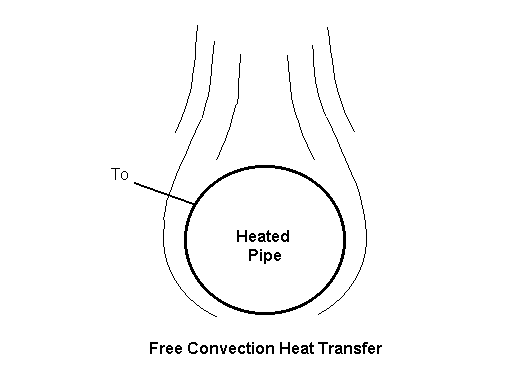Heat Loss by Free Convection
Ceng 402 Project

Introduction

Free convection is a limiting case of convection in which heat is transported upward by heated fluid which rises. The flow patterns are determined by the buoyancy effect of the heated fluid, as opposed to being determined by some external force as in forced convection. The following correlations are useful for estimating heat losses from various structures. It is assumed that the temperature is constant at T0 on the surface of the object and at Tinf far from the surface. The fluid properties such as density, rho, and viscosity, mu, are to be evaluated at the mean temperature, or film temperature

Tf = (T0 + Tinf) / 2

There are several dimensionless parameters used in calculations involving heat transfer by convection. The Nusselt number Num for free-convection heat transfer for an object submerged in an infinite fluid is of the form

Num = Nu (Gr Pr)

in which Num is based on the heat-transfer coefficient hm for the total surface of the submerged object

Num = hm D / k

where D is the diameter, and k is the thermal conductivity.

The Grashof number Gr is another dimensionless parameter

Gr = rho2 beta g D3 (T0 - Tinf) / mu2

where beta is 1 / Tf for ideal gases, g is the gravitational acceleration.

The dimensionless Prandlt number Pr is given by

Pr = Cp mu / k

where Cp is the specific heat of the fluid.

For a single sphere of diameter D in a large body of fluid, when Gr1/4Pr1/4 < 200, the Nusselt number is closely represented by

Num = 2 + 0.59 Gr1/4 Pr1/4

For a long horizontal cylinder in an infinite fluid when GrPr > 104, the Nusselt number is closely represented by

Num = 0.518 (Gr Pr)1/4

Diagram:
Free Convection from a Horizontal Cylinder